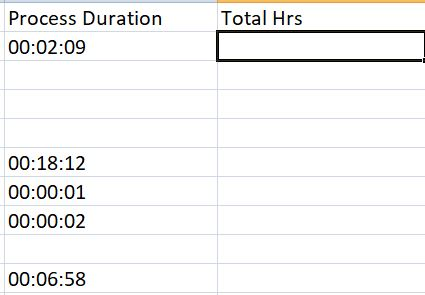# How to sum up times in an excel, taking the last cell with time as dynamic

Hello everyone,

I wanted to sum up all the times in the given column in excel and the number of counts in the data table is dynamic, so as the last time column.
I tried getting count of the table and then passing it in the formula, but it’s not working right. Attaching a sample image. Need to add all the time in process duration and sum up in a cell in Total hours.
=SUM(E2:E10) isn’t working for me, it’s giving just last cell time, what’s working is =E2+E3+…+E10
Any suggestions would be appreciated.
ThanksHi,

Your data in E2:E9 seems not datetime type but string type.
So can you try the following?

``````new TimeSpan(dt.AsEnumerable.Select(Function(r) r("Process Duration").ToString).Where(Function(t) TimeSpan.TryParse(t,New TimeSpan)).Sum(Function(ts)  TimeSpan.Parse(ts).Duration.Ticks)).ToString
``````

Regards,

2 Likes

It is because the value at E10 (at least) is not a datetime format and that is why it is giving this last value. An easy remedy is to format all relevant cells to datetime format or take guidance from the image attached. You will hopefully get the desired result.1 Like

Thanks @J_Malik and @Yoichi for your response.
I did checked with Format cells. It’s in this form

And was trying to sum up this way

But it’s giving output in total hours as 00:00:00

check this post

Thanks for responding. This solution is showing me this

Though first time is printed correctly in the message box. But I need to find the sum of all the times.

I am wondering on “=E2+E3+E4+E5+…+E10” is working fine, but why “=SUM(E2:E10)” is giving output as 00:00:00??
Since I couldn’t apply the formula for dynamic no. of rows in first one

variable time is GenericValue, we need to convert that to TimeSpan type and then use .Add() method to add the time

more details

after Format value activity

• create a variable of System.TimeSpan type, lets say TempTime
• assign TempTime = TimeSpan.Parse(time) this converts the GenericValue of time to TimeSpan type
• create another variable lets say total_time of TimeSpan type, assing value 0 before the For Each Row. Now within the for loop assing total_time = total_time.Add(TempTime) this will add all the times in the excel

Hope this helps. If this answers you please mark as solution so it helps others with similar doubt

1 Like

Is it possible for you to share sample file for the task you are performing with one of the dynamic value?

Reformat F2 as follows and if there is no issue with source data then that is it.1 Like

Hi @J_Malik

@AdityaVN , this is working for me